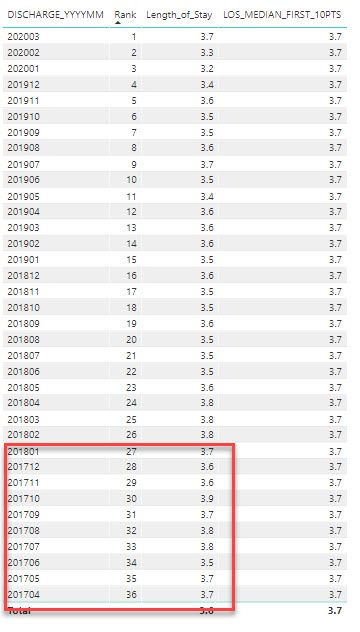cancel
Showing results for
Did you mean:Frequent Visitor

## Get MEDIAN for first 10 months of three years of data

Hello,

My requirement is to pull three years of data from today, the ask is to display length of stay over this three years of data by month (YYYYMM) in a line chart.  In addition, add MEDIAN length of stay as a dash line, the calculation for MEDIAN is only take median of the first 10 starting months of data as circled on 2nd table screenshot.To do this, I created a RANK column below as shown on the above table:

Rank = rankx(ALLSELECTED(eras), ERAS[DISCHARGE_YYYYMM],,DESC,dense)

And add LOS_MEDIAN_FIRST_10PTS measure below as shown on the above table:
LOS_MEDIAN_10PTS = CALCULATE(MEDIANX(ERAS, AVERAGE(ERAS[LOS_EST_DAYS])), ERAS[Rank]>=27 && ERAS[Rank] <=36 , ALL(ERAS[DISCHARGE_YYYYMM]))

This appears to be working fine for initial requirement. However, the new requirement comes in and this is how the problem starts.
If I add a DATE slicer (users can choose any dates), my rank column doesn't start from rank = 1.
It displays oringal rank, not dynamically. So my LOS_MEDIAN_10PTS measure logic above will not work.I tried to add RANK measure below, and this measure ranks correctly starting from rank 1 and count up.
RANK_TEST = RANKX ( ALLSELECTED ( ERAS[DISCHARGE_YYYYMM] ),
CALCULATE ( SELECTEDVALUE ( ERAS[DISCHARGE_YYYYMM] ) ),
,
ASC, Dense
)

However, I couldn't add this measure to replace a rank filter in red below.

LOS_MEDIAN_10PTS = CALCULATE(MEDIANX(ERAS, AVERAGE(ERAS[LOS_EST_DAYS])), [RANK2]>=1 && [RANK2]<=10 , ALL(ERAS[DISCHARGE_YYYYMM]))

1 ACCEPTED SOLUTIONCommunity Support

Please create a measure as below to work on it instead of using a calculated column.

``````Measure =
VAR mindate =
CALCULATE ( MIN ( 'ERAS'[date] ), ALLSELECTED ( ERAS ) )
VAR maxdate =
EDATE ( mindate, 10 )
RETURN
CALCULATE (
AVERAGE ( ERAS[Length_of_Stay] ),
FILTER ( ALL ( ERAS ), 'ERAS'[date] >= mindate && 'ERAS'[date] < maxdate )
)
``````For more details, please check the pbix as attached.

Community Support Team _ Frank
If this post helps, then please consider Accept it as the solution to help the others find it more quickly.
6 REPLIES 6Community Support

Please create a measure as below to work on it instead of using a calculated column.

``````Measure =
VAR mindate =
CALCULATE ( MIN ( 'ERAS'[date] ), ALLSELECTED ( ERAS ) )
VAR maxdate =
EDATE ( mindate, 10 )
RETURN
CALCULATE (
AVERAGE ( ERAS[Length_of_Stay] ),
FILTER ( ALL ( ERAS ), 'ERAS'[date] >= mindate && 'ERAS'[date] < maxdate )
)
``````For more details, please check the pbix as attached.

Community Support Team _ Frank
If this post helps, then please consider Accept it as the solution to help the others find it more quickly.Frequent Visitor

That helps. Thanks so much!Super User

See if this way Rank can help

new Rank =
var _min = mix(allselected(ERAS),[Rank])
return
[Rank]-_min +1Frequent Visitor

Thanks atmitchandak for a quick reply!

I created a new rank as column and I've received this error "The expression refers to mutiple columns.  Multiple columns cannot be converted to scalar value."

new Rank =

var _min = min(allselected(ERAS), ERAS[Rank])

return

ERAS[Rank]-_min +1Frequent Visitor

Oh, there was a typo in MINX, it should be

new Rank =

var _min = minx(allselected(ERAS), ERAS[Rank])

return

ERAS[Rank]-_min +1

However, the new rank value still look same as the old rank. It doens't dynamically re-rank.Super User

Become an expert!: Enterprise DNA
External Tools: MSHGQM
Latest book!:
Mastering Power BI 2nd EditionDAX is easy, CALCULATE makes DAX hard...